﻿ GRAPES新云量计算方案的引进和影响试验
 快速检索 中文标题 英文标题 作者中文名 作者英文名 单位中文名 单位英文名 中文关键词 英文关键词 中文摘要 英文摘要 基金项目 全文
 气象2013, Vol. 39Issue (1): 57-66.  DOI: 10.7519/j.issn.1000-0526.2013.01.007### 引用本文 [复制中英文]

[复制中文]
ZHENG Xiaohui, XU Guoqiang, WEI Rongqing, 2013. Introducing and Influence Testing of the New Cloud Fraction Scheme in the GRAPES[J]. Meteorological Monthly, 39(1): 57-66. DOI: 10.7519/j.issn.1000-0526.2013.01.007.
[复制英文]

### 文章历史

2011年12月29日收稿
2012年8月03日收修定稿
GRAPES新云量计算方案的引进和影响试验

1. 中国气象科学研究院，北京 100081
2. 国家气象中心，北京 100081
3. 新疆维吾尔自治区气象台，乌鲁木齐 830002

Introducing and Influence Testing of the New Cloud Fraction Scheme in the GRAPES
ZHENG Xiaohui1, XU Guoqiang2, WEI Rongqing3
1. Chinese Academy of Metorological Sciences, Beijing 100081;
2. National Meteorological Centre, Beijing 100081;
3. Meteorological Observatory of Xinjiang Uygur Autonomous Region, Urumqi 830002
Abstract: By numerical experiments, the EC cloud fraction schemes (ECFS), the WRF cloud fraction schemes (WCFS), the GRAPES cloud fraction scheme (GCFS) and the simple cloud fraction scheme (SCFS) are compared. The results show that: (1) The cloud coverage is well simulated by all schemes, but the results by ECFS and SCFS are more accurate; (2) The total cloudiness simulation is more than observations in SCFS, that in GCFS and WCFS is less than observations, and that in ECFS is closer to observations; (3) The surface temperature is general agreement between simulations and observations in East China, but the temperature has much error in West China. By contrast, the simulation in ECFS is closer to observations; (4) Based on a comprehensive analysis, ECFS produces a better simulation. Thus ECFS can be used as a reference of a new cloud fraction scheme which would be introduced into GRAPES model.
Key words: GRAPES model    cloud fraction scheme    numerical experiment

1 GRAPES模式与云量计算方案简介 1.1 GRAPES模式简介

GRAPES(Global/Regional Assimilation and Prediction Enhanced System)模式是我国自主研发的一个多尺度通用模式，兼顾全球与有限区可选的预报区域设计，静力与非静力可选的平衡设计，采用半隐式-半拉格朗日时间差分方案，经纬度格点的网格设计，水平方向取Aracawa C格点，垂直方向采用Charney-Philips格式非均匀跳层变量配置，高度为地形追随坐标。模式的物理过程包括积云对流过程、微物理过程、辐射过程、边界层过程和陆面过程等(陈德辉等，2008薛纪善等，2008)。

1.2 云量计算方案简介 1.2.1 GRAPES模式现用云量计算方案

GRAPES模式现用云量计算方案(下文中简称GRAPES云方案)是云量诊断方案，体现相对湿度与云量之间的关系。首先计算出水面和冰面的饱和混合比。再根据温度区分云水和云冰，确定云水和云冰的比例系数faf(式1)，并根据该比例系数计算云水、云冰的饱和比湿和rqs(式2)：

 $\left\{ \begin{array}{l} \mathit{faf}{\rm{ = 0}}{\rm{.0}}\;\;\;\;\;\;\;\;\;\;\;\;\;\;\;\mathit{t} \ge {\rm{273}}{\rm{.15}}\\ \mathit{faf}{\rm{ = }}\frac{{{\rm{273}}{\rm{.15 - }}\mathit{t}}}{{{\rm{40}}}}\;\;\;\;\;\;{\rm{273}}{\rm{.15 > }}\mathit{t}{\rm{ > 233}}{\rm{.15}}\\ \mathit{faf}{\rm{ = 1}}{\rm{.0}}\;\;\;\;\;\;\;\;\;\;\;\;\;\;\;{\rm{233}}{\rm{.15}} \ge \mathit{t} \end{array} \right.$ (1)
 $\mathit{rqs}{\rm{ = }}\left({{\rm{1 - }}\mathit{faf}} \right) \times {\mathit{Q}_{{\rm{vsw}}}}{\rm{ + }}\mathit{faf} \times {\mathit{Q}_{{\rm{vsi}}}}$ (2)

 $\mathit{RH}{\rm{ = min}}\left[ {{\rm{1}}{\rm{.0, }}\frac{{{\mathit{Q}_\mathit{v}}}}{{\mathit{rqs}}}} \right]$ (3)
 $\begin{array}{l} \mathit{cc}{\rm{ = min}}\left\{ {{\rm{1}}{\rm{.0, }}\left[ {{\rm{1}}{\rm{.0 - exp}}\left({ - \frac{{{\rm{100(}}{\mathit{Q}_\mathit{c}}{\rm{ + }}{\mathit{Q}_\mathit{i}}{\rm{)}}}}{{{\mathit{Q}_{{\rm{vsw}}}}}}} \right)} \right]} \right. \cdot \\ \;\;\;\;\;\;\left. {{\rm{exp}}\left[ {{\rm{0}}{\rm{.25ln}}\left({\mathit{RH}} \right)} \right]} \right\} \end{array}$ (4)
 ${\mathit{C}_\mathit{f}}{\rm{ = min}}\left[ {{\rm{1}}{\rm{.0, }}\mathit{cc}\left({{\rm{1 + 2}}{\mathit{Q}_{{\rm{vsi}}}}} \right)} \right]$ (5)

1.2.2 WRF模式现用云量计算方案

WRF模式现用的云量计算方案(下文中简称WRF云方案)也是一种云量诊断方案，在计算流程上与GRAPES云方案类似。不同的是在WRF云方案中，引入了对雪的判断，故在区分云水、云冰和雪是分为两种情况：(a)当云水、云冰和雪都存在时，如式(6)，其中Qc为云水混合比，Qi为云冰混合比，Qs为云雪混合比；(b)只有云水存在时，如式(7)。

 $\left\{ \begin{array}{l} \mathit{faf}\;\;{\rm{ = }}\;\;{\rm{0}}\\ \;\;\;\;\;\;\;\;{\mathit{Q}_i} + {\mathit{Q}_\mathit{c}} + {\mathit{Q}_s} < {10^{ - 12}}{\rm{kg}} \cdot {\rm{k}}{{\rm{g}}^{ - 1}}\\ \mathit{faf}\;\;{\rm{ = }}\;\;\frac{{{\mathit{Q}_i} + {\mathit{Q}_s}}}{{{\mathit{Q}_i} + {\mathit{Q}_s} + {\mathit{Q}_c}}}\\ \;\;\;\;\;\;\;{\mathit{Q}_i} + {\mathit{Q}_s} + {\mathit{Q}_c} \ge {10^{ - 12}}{\rm{kg}} \cdot {\rm{k}}{{\rm{g}}^{ - 1}} \end{array} \right.$ (6)
 $\left\{ \begin{array}{l} \mathit{faf}\;\;{\rm{ = }}\;\;{\rm{0}}\\ \;\;\;\;\;\;\;\;{\mathit{Q}_\mathit{c}} < {10^{ - 12}}{\rm{kg}} \cdot {\rm{k}}{{\rm{g}}^{ - 1}}\\ \mathit{faf}\;\;{\rm{ = }}\;\;{\rm{0}}\\ \;\;\;\;\;\;\;{\mathit{Q}_\mathit{c}} \ge {10^{ - 12}}{\rm{kg}} \cdot {\rm{k}}{{\rm{g}}^{ - 1}}并且t > 273.15{\rm{K}}\\ \mathit{faf}\;\;{\rm{ = }}\;\;1\\ \;\;\;\;\;\;\;{\mathit{Q}_\mathit{c}} \ge {10^{ - 12}}{\rm{kg}} \cdot {\rm{k}}{{\rm{g}}^{ - 1}}并且t \le 273.15{\rm{K}} \end{array} \right.$ (7)

 $\mathit{RH}{\rm{ = max}}\left[ {{\rm{1}}{{\rm{0}}^{ - 10}}{\rm{, }}\frac{{{\mathit{Q}_\mathit{v}}}}{{\mathit{rqs}}}} \right]$ (8)
 $\arg = \max \left[ { - 6.9, - \frac{{100{\mathit{Q}_\mathit{c}}}}{{\max \left({{\rm{1}}{{\rm{0}}^{ - 10}}{\rm{, }}\mathit{rqs} - {\mathit{Q}_\mathit{v}}} \right)}}} \right]$ (9)
 $c_f = \frac{{R{H^b}}}{a}\left[ {1 - \exp \left({\arg } \right)} \right]$ (10)

1.2.3 ECMWF云量计算方案

EC模式的云量计算包括预报和诊断两种方案，在本文中为了保证4种云方案对比的一致性，选取EC诊断云方案进行说明和对比。

 ${C_c} = a + b\ln \mathit{P}$ (11)

 ${C_f} = rc \times {C_c} + {\left({\frac{{rh - crh}}{{1 - crh}}} \right)^2} \times w \times rl$ (12)

1.2.4 简单云量计算方案

 $\left\{ \begin{array}{l} {\mathit{Q}_\mathit{c}} + {\mathit{Q}_i} > {10^{ - 6}}{\rm{kg}} \cdot {\rm{k}}{{\rm{g}}^{ - 1}}\;\;\;\;\;{C_f} = 1\;\;\;有云\\ {\mathit{Q}_\mathit{c}} + {\mathit{Q}_i} \le {10^{ - 6}}{\rm{kg}} \cdot {\rm{k}}{{\rm{g}}^{ - 1}}\;\;\;\;\;{C_f} = 0\;\;\;无云 \end{array} \right.$ (13)
1.3 WSM6云微物理方案简介

2 资料与数值试验方案设计 2.1 资料

2.2 数值试验方案设计

3 数值试验结果分析 3.1 云分布模拟对比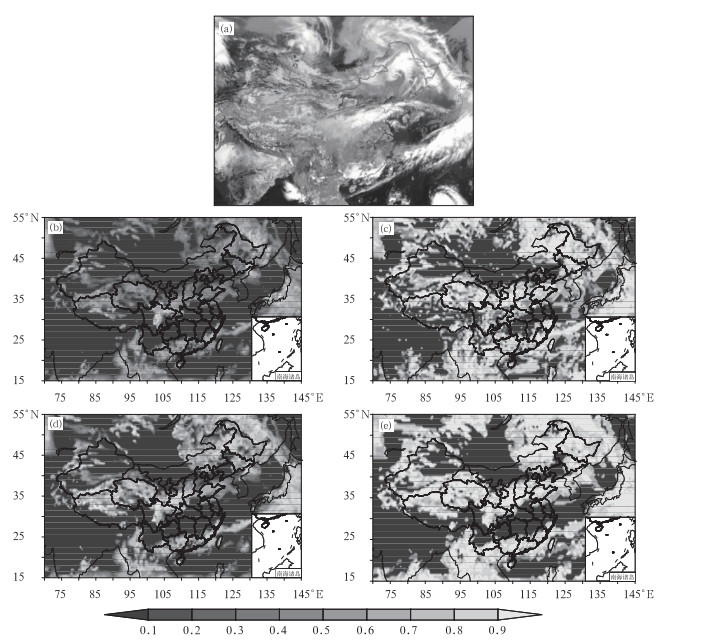图 1 2008年5月29日06时云分布 (a)风云二号卫星可见光云图, (b)GRAPES云方案, (c)EC云方案, (d)WRF云方案, (e)简单云方案 Fig. 1 The cloud coverages at 06:00 UTC 29 May 2008 from (a) VIS satelite image of FY-2, (b) GCFS, (c) ECFS, (d) WCFS, and (e) SCFS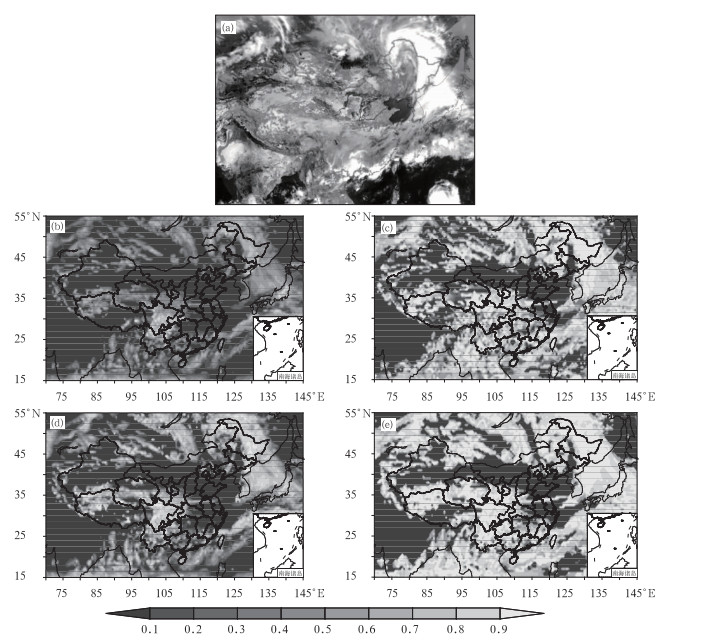图 2 2008年5月30日06时云分布 (a)风云二号卫星可见光云图，(b)GRAPES云方案，(c)EC云方案，(d)WRF云方案，(e)简单云方案 Fig. 2 The cloud coverages at 06:00 UTC 30 May 2008 from (a) VIS satelite image of FY-2, (b) GCFS, (c) ECFS, (d) WCFS, and (e) SCFS
3.2 云量模拟对比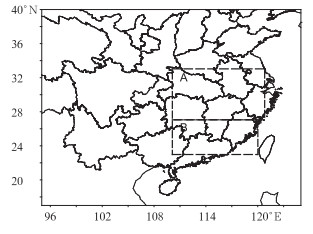图 3 选择的对比区域 (A:长江中下游地区，B:华南地区) Fig. 3 Contrast areas A and B (A: The middle and lower reaches of Yangtze River, B: South China)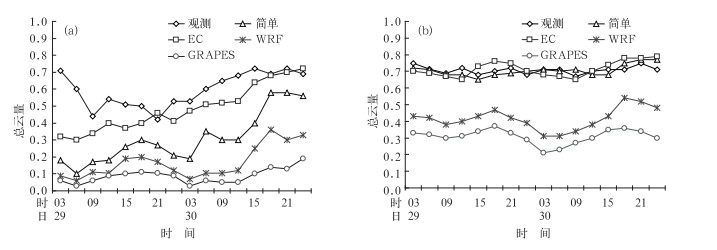图 4 2008年5月29日03时至31日00时总云量随时间的变化 (a)长江中下游地区，(b)华南地区 Fig. 4 The evolution of total cloud fraction (03:00 UTC 29-00:00 UTC 31, May 2008) in (a) the middle and lower reaches of Yangtze River, and (b) South China

3.3 温度场模拟对比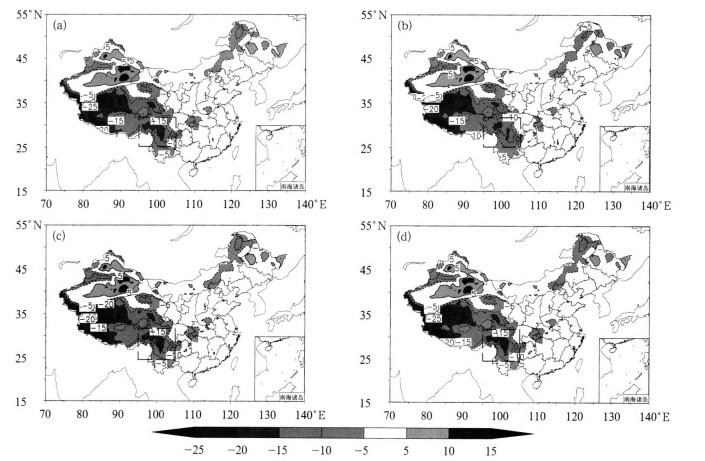图 5 2008年5月30日00时模拟的地面温度与观测的地面温度的差值分布(单位：℃) (a)GRAPES-观测，(b)EC-观测，(c)WRF-观测，(d)简单-观测 (虚线框为采用不同云量方案的模拟结果) Fig. 5 The difference distribution between observed and simulated surface temperatures at 00:00 UTC 30 May 2008 (unit:℃) (a) GCFS-Obs, (b) ECFS-Obs, (c) WCFS-Obs, (d) SCFS-Obs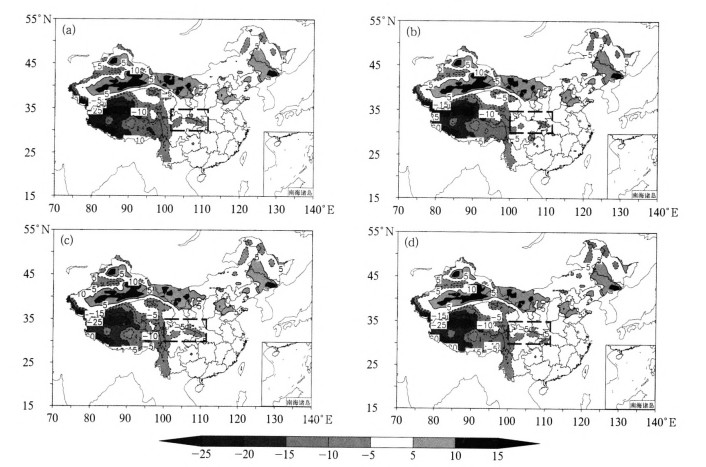图 6 2008年5月31日00时模拟的地面温度与观测的地面温度的差值分布(单位：℃) (a)GRAPES-观测，(b)EC-观测，(c)WRF-观测，(d)简单-观测 Fig. 6 As in Fig. 5, but for 00:00 UTC 31 May 2008 (a) GCFS-Obs, (b) ECFS-Obs, (c) WCFS-Obs, (d) SCFS-Obs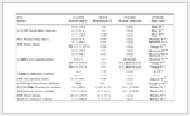表 1 全国和区域范围内观测与模拟的地面温度差值(单位：℃)
(区域表示上文中的虚线框范围，全国表示全国范围)
Table 1 The difference between observed and simulated surface temperatures in national and regional scale (unit: ℃)
(Regional shows the dotted box in the above, national shows nationwide)

4 小结

(1) 通过模拟的云分布状况与风云二号卫星可见光云图的对比，表明4种云量计算参数化方案都能大致模拟出云带的分布，但EC与简单云方案模拟的云分布更为准确，WRF和GRAPES云方案模拟的云分布区域偏小。

(2) 通过模拟的总云量与地面观测云量随时间变化的对比，表明GRAPES和WRF云方案模拟的总云量偏少，EC和简单云方案模拟的总云量较为接近观测值。

(3) GRAPES模式采用4种不同云量计算方案模拟出的地面温度场与观测值相对比，在中国东部地区模拟的地面温度与观测基本接近，但在中国西部地区则误差较大；从全国和局部区域的地面温度平均值对比中发现，采用EC云方案时模式模拟的地面温度更接近观测值。

(4) 通过在GRAPES模式中采用不同云量计算方案进行数值试验，综合分析模拟结果与观测值的对比效果，认为EC云量计算方案模拟的效果最佳，可以作为一种新的云量计算方案引进GRAPES模式中。

 陈德辉, 薛纪善, 杨学胜, 等, 2008. GRAPES新一代全球/区域多尺度统一数值预报模式总体设计研究[J]. 科学通报, 53(20): 2396-2407. DOI:10.3321/j.issn:0023-074X.2008.20.002 戴福山, 宇如聪, 张学洪, 等, 2004. 一个统计低云方案及其在大气环流模式中应用初探[J]. 气象学报, 62(4): 385-394. DOI:10.11676/qxxb2004.039 钱云, 1994. 区域气候模式中云量参数化方案的研究[J]. 热带气象学报, 10(4): 342-348. 石广玉, 2007. 大气辐射学[M]. 北京: 科学出版社, 134-135. 王咏青, 张楠, 刘敏, 等, 2010. 大气模式中云量诊断的系统性误差研究[J]. 气候与环境研究, 15(1): 83-88. 薛纪善, 陈德辉, 2008. 数值预报系统GRAPES的科学设计与应用[M]. 北京: 科学出版社, 331-342. 徐国强, 陈德辉, 薛纪善, 等, 2008. GRAPES物理过程的优化试验及程序结构设计[J]. 科学通报, 53(20): 2428-2434. DOI:10.3321/j.issn:0023-074X.2008.20.006 徐兴奎, 2012. 中国区域总云量和低云量分布变化[J]. 气象, 38(1): 90-95. DOI:10.7519/j.issn.1000-0526.2012.01.010 闫之辉, 王雨, 朱国富, 2010. 国家气象中心业务数值预报发展的回顾与展望[J]. 气象, 36(7): 26-32. DOI:10.7519/j.issn.1000-0526.2010.07.007 章建成, 刘奇俊, 2006. GRAPES模式不同云物理方案对短期气候模拟的影响[J]. 气象, 32(7): 3-12. DOI:10.7519/j.issn.1000-0526.2006.07.001 Gu Y, Farrara J, 2003. Parameterization of cloud-radiation processes in the UCLA general circulation model[J]. J Climate, 16(2): 3357-3370. Teixeira J, 2001. Cloud fraction and relative humidity in a prognostic cloud fraction scheme[J]. Mon Wea Rev, 129(7): 1750-1753. DOI:10.1175/1520-0493(2001)129<1750:CFARHI>2.0.CO;2 Tiedtke M, 1993. Representation of clouds in large-scale models[J]. Mon Wea Rev, 121(11): 3040-3058. DOI:10.1175/1520-0493(1993)121<3040:ROCILS>2.0.CO;2 Tompkins A M, 2002. A prognostic parameterization for the subgrid-scale variability of water vapor and clouds in large-scale models and its use to diagnose cloud cover[J]. J Atmos Sci, 59(12): 1917-1942. DOI:10.1175/1520-0469(2002)059<1917:APPFTS>2.0.CO;2 Slingo J M, 1987. The development and verification of a cloud prediction scheme for the ECMWF model[J]. Quart J R Met Soc, 113(447): 899-927. Sommeria G, 1977. Subgrid-scale condensation in models of nonprecipiting clouds[J]. J Atmos Sci, 34(2): 344-355. DOI:10.1175/1520-0469(1977)034<0344:SSCIMO>2.0.CO;2